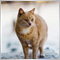• Overview
• Reviews (1)

# BandWidth Squeeze tfmt4

This indicator lets you customize your own squeeze criteria to see when the Bollinger Bands squeeze and when the bands start expanding again. BandWidth is the numerical difference between the upper and lower Bollinger Bands. This indicator shows the BandWidth line and a squeeze or threshold line that is the lowest BandWidth plus a percentage you specify. The squeeze line is in yellow. The BandWidth that is below the squeeze line is in green and the BandWidth that is above the squeeze line is in red. As you calculate your squeeze line, you can use this indicator to see when the price may begin to trend along one of the Bollinger Bands as the volatility increases.

The inputs let you change to a tight or loose squeeze line by specifying a percentage of the lowest BandWidth to add to the lowest BandWidth. The inputs also let you change the number of bars to look back and find the lowest BandWidth value. Use together with the Bollinger Bands indicator that comes with your installation to view the bands contract and expand. The inputs include:

• Band_Periods - The number of bars used in the SMA to create the Bollinger Bands for the BandWidth calculation.
• Deviations - The number of standard deviations used to create the Bollinger Bands for the BandWidth calculation.
• BandWidth_Bars - The number of bars back to find the lowest BandWidth value.
• BandWidth_Percent - The percentage to add to the lowest BandWidth value. If you want the threshold line to be 20% above the lowest BandWidth value, enter 20 to this input.

BandWidth Squeeze/Threshold Line summarized calculation:

Lowest BandWidth of BandWidth_Bars + ( Lowest BandWidth of BandWidth_Bars * BandWidth_Percent )8576
2018.12.16 04:57

Thanks for sharing